# IFS Function

Returns a value that meets the first TRUE condition

## What is the IFS Function?

The IFS Function in Excel is a Logical function that was introduced in Excel 2016. The function is an alternative to the Nested IF function and is much easier to use. The IFS function checks if one or more than one conditions are observed or not and accordingly returns a value that meets the first TRUE condition.

### Formula

= IFS(logical_test1, Value1 [logical_test2, Value2] …, [logical_test127, Value127])

Where:

1. Logical_test1 – First logical test. This is a required argument and is the condition that is used by Excel to evaluate whether it is TRUE or FALSE.
2. Value1 – Result when logical_test1 is true. If required, you can keep it empty.

The rest of the logical_test and Value arguments are optional. The function allows the user to put in up to 127 logical_test arguments using the IFS function.

### How to use the IFS Function in Excel?

It is a built-in function which can be used as a worksheet function in Excel. Let’s take a look at an example:

Assume we wish to assign grades to marks earned by students. We can use the IFS Function in the following manner: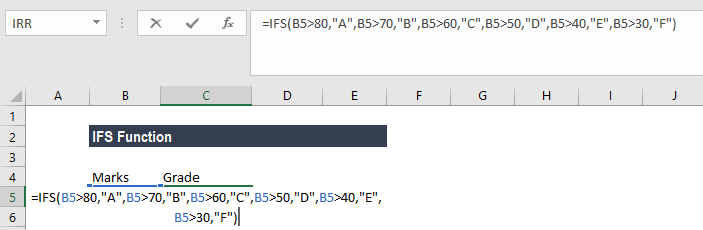The formula used is:

IFS(A2>80,”A”,A2>70,”B”,A2>60,”C”,A2>50,”D”,A2>40,”E”,A2>30,”F”), which says that if cell A2 is greater than 80 then return an “A” and so on.

Using this formula, the result would be: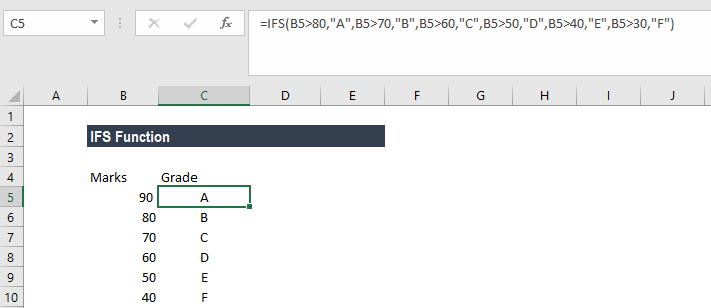### Examples of the IFS Function in Excel

To understand the uses of this function, let’s consider a few more examples:

#### Example 1 – Using IFS with ELSE

Assume we have a list of items and we need to classify them under four common headings: Vegetable, Fruit, Green Vegetable, and Beverage. When we use the IFS function and give the formula =IFS(A2=”Apple”,”Fruit”,A2=”Banana”,”Fruit”,A2=”Spinach”,”Green Vegetable”,A2=”coffee”,”Beverage”,A2=”cabbage”,”Green Vegetable”,A2=”capsicum”,”Vegetable”)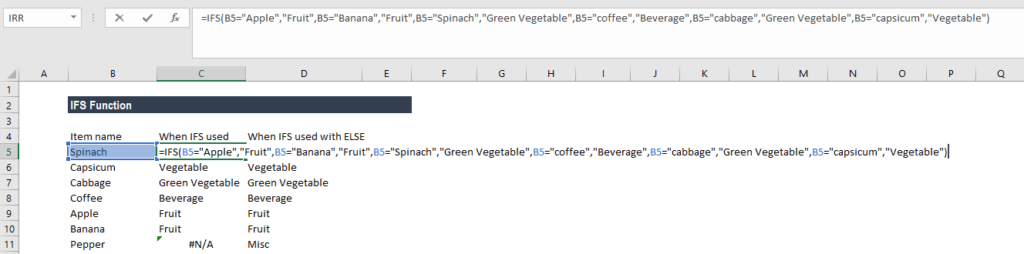Then, we will get the following result: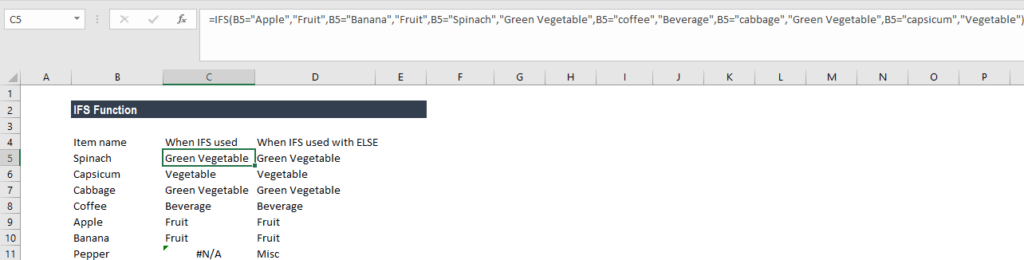In this example, we have entered multiple logical tests in the IFS function. When a logical test evaluates to TRUE, the corresponding value will be returned. However, if none of the logical tests evaluate to TRUE, then IFS function would give #N/A error. This is what we got for Pepper in B8.

Using the ELSE function, we can ensure that this #N/A error is removed. For this, we would place a final logical test at the end of the formula that is TRUE and then place a value that shall be returned.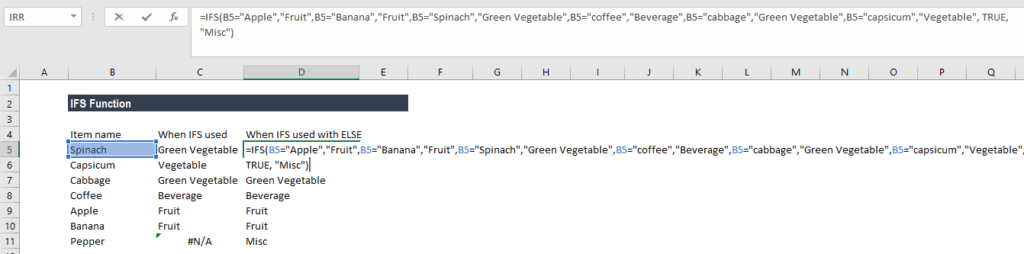Here, we added TRUE, “Misc”, this will ensure that Excel returns the value “Misc” in the scenario where none of the previous logical tests in the IFS function evaluates to TRUE.

Now the results would as be as shown below: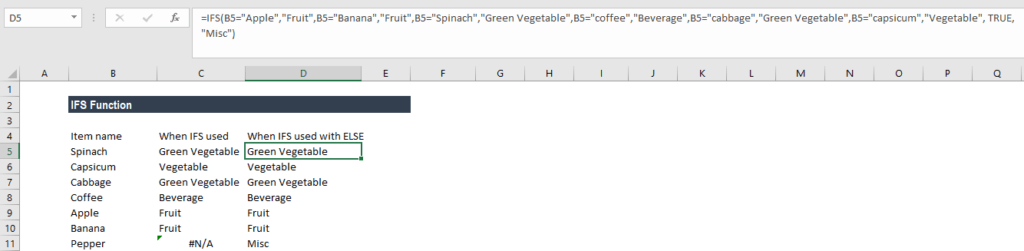#### Example 2 – IFS vs nested IF function

Prior to the introduction of the IFS function in Excel, we used to use nested IF function. Let’s see how the IFS function is superior to nested IF. Let’s assume we wish to give discounts to customers based on their total purchases from us. So, a customer would get a 5% discount for purchases more than \$100 but less than \$500, a 10% discount if he made purchases of more than \$500 but less than \$750, 20% if he made purchases of more than \$750 but less than \$1,000, and 30% for all purchases above \$1,000.

Let’s see the formula when we would have used nested IF function: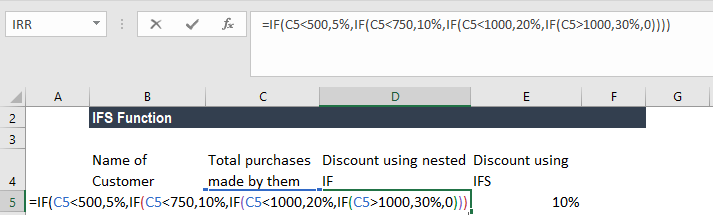Now, let’s see how it becomes easy to write the formula for same results using IFS: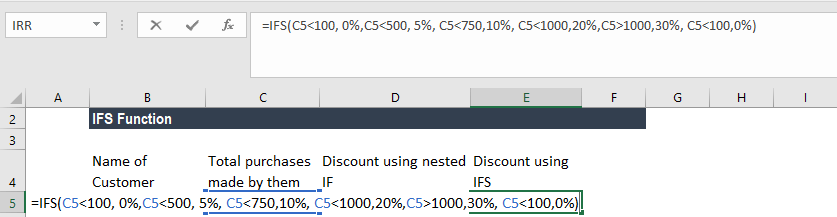Hence, IFS is easier, as it allows using a single function to input a series of logical tests. It becomes cumbersome in the nested IF function, especially when we use a large number of logical tests.

### A few pointers on the IFS function

1.  #N/A Error occurs when no TRUE conditions are found by IFS function.
2. #VALUE! Error – We will get this error when the logical_test argument resolves to a value other than TRUE or FALSE.
3. “You’ve entered too few arguments for this function” error message – This message appears when we provide a logical_test argument without a corresponding value.

Thanks for reading CFI’s guide to the IFS function in Excel. By taking the time to learn and master Excel functions, you’ll significantly speed up your financial analysis. To learn more, check out these additional CFI resources:

• Excel Functions for Finance